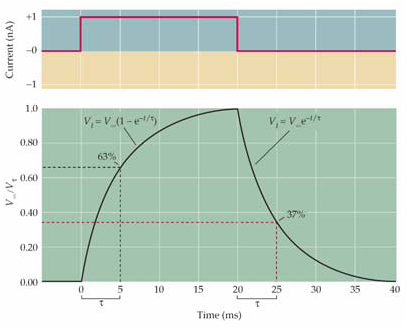# Thread: Values in GoldmanHodgkinKatz Equation ?

1. See pdf: http://dsc.ing.ula.ve/dokuwiki/_medi..._2010_cap1.pdf

I need to modeling the 1.12 equation (page 10) in Matlab, it corresponds to the circuit you can see up (for the membrane potential). I need numerical values of Cm (neuronal membrane capacitance), Gcl, Gk, Gna (chlorine, pottasium and sodium conductance), Ecl, Ena, Ek (chlorine, pottasium and sodium batteries) and I(t)/A is a step signal (I want to know this value too).

This equation models the membrane potential in a neuron.

I'm a engineer systems student (don't speak english), thats because I dont know a lot about it. The Goldman–Hodgkin–Katz works for a failed potential, it means is not a action potential, only works for a hyperpolarization and depolarization between -70 and -55 mV

I used tentative (crazy) values and the following formula in matlab:

di(1)= -(Gcl/Cm)*(i(1)-Ecl) -(Gk/Cm)*(i(1)-Ek) -(Gna/Cm)*(i(1)-Ena) + I;

I=-60;

Cm = 0.9;
Gcl = 0.9;
Gk =0.9;
Gna =0.9;
Ecl =0.9;
Ek =0.9;
Ena =0.9;

And find this:logically is wrong, the rest pontential have to be -70, -65 mV.

When I find values, then, changing the I(t)/A signal ..I have to find something like this, but the second graphic negative.Thank you if you can help me.2.

 matlab, membrane cell, neuroscience, physiology, potential